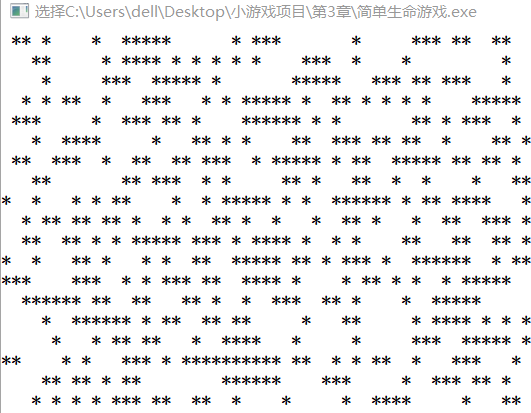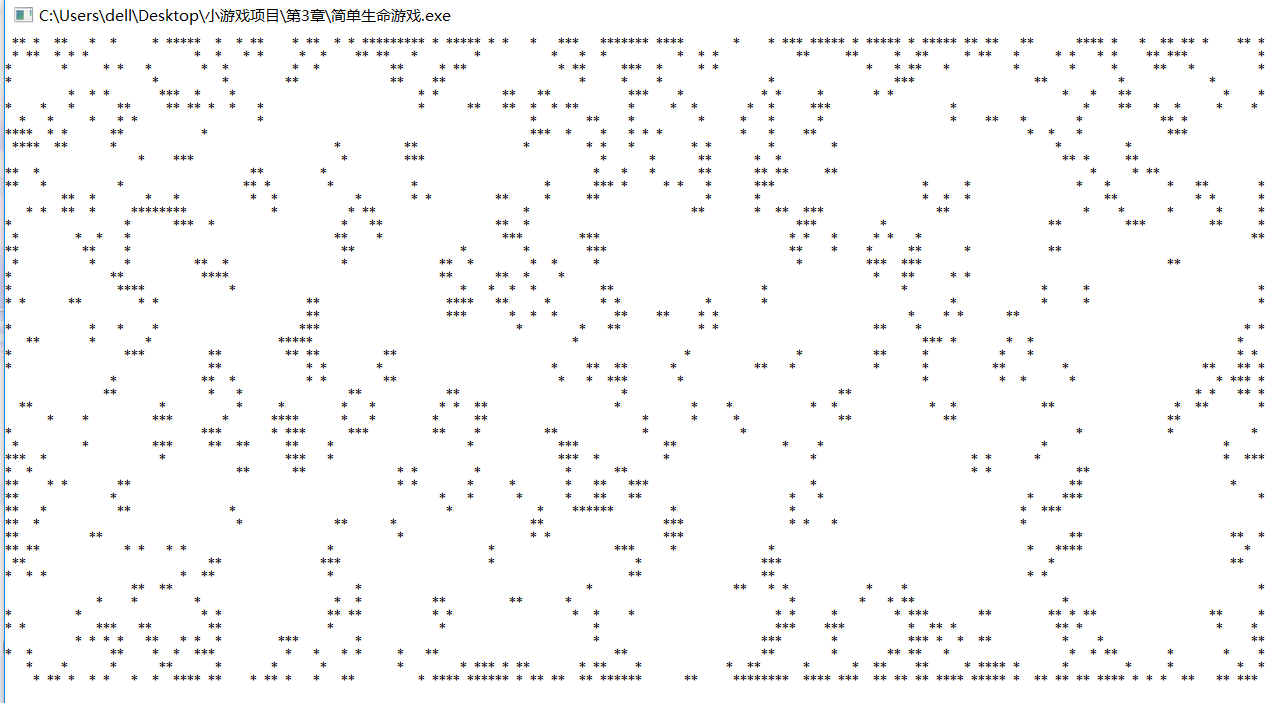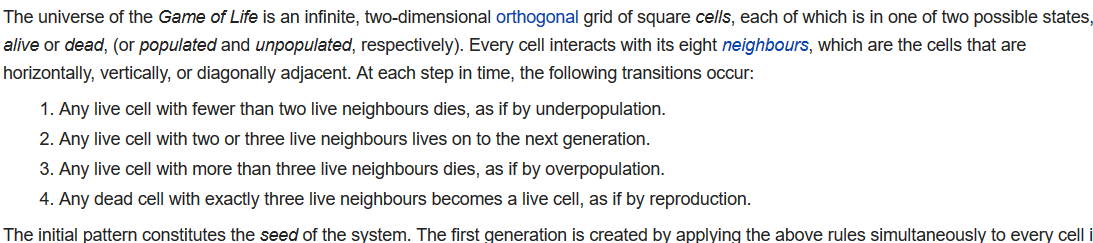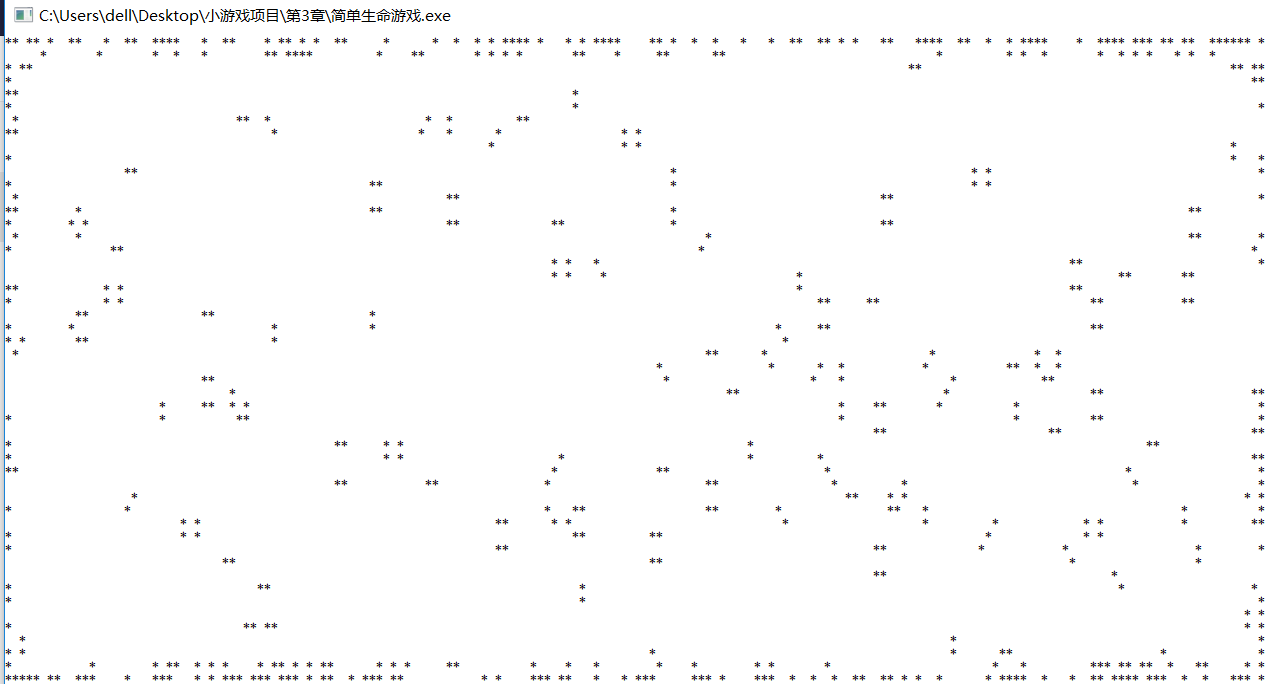# C/C++实现简单生命游戏(Game Of Life)

## 点子-Game of Life

1． 如果一个细胞周围有3个细胞为生，则该细胞为生（即该细胞若原先为死，则转为生，若原先为生，则保持不变）;
2． 如果一个细胞周围有2个细胞为生，则该细胞的生死状态保持不变；
3． 在其它情况下，该细胞为死（即该细胞若原先为生，则转为死，若原先为死，则保持不变）。

## 实现

### 1. 游戏框架-静止图案

#include <stdio.h>
#include <windows.h>
#include <time.h>
#include <stdlib.h>
#include <conio.h>

#define Height 30 //游戏画面尺寸
#define Width 50
int Cells[Height][Width]; //所有位置随机置1或0

void startup() {
for (int i = 0; i < Height; ++i) {
for (int j = 0; j < Width; ++j) {
Cells[i][j] = rand() % 2;
}
}
}
void show() {
for (int i = 0; i < Height; ++i) {
for (int j = 0; j < Width; ++j) {
if (Cells[i][j]) printf("*"); //活的细胞输出
else printf(" ");             //死的细胞为空
}
printf("\n");
}
}
void updateWithoutInput() { //游戏内部更新

}
void updateWithInput() { //用户输入相关更新

}

int main() {
srand(time(NULL));
startup();
while (1) {
show();
updateWithoutInput();
updateWithInput();
}
return 0;
}### 2. 动态图案-按规则繁衍死亡

(i - 1, j - 1)(i - 1, j)(i - 1, j + 1)
(i, j - 1)(i, j)(i, j + 1)
(i + 1, j - 1)(i + 1, j)(i + 1, j + 1)

#include <stdio.h>
#include <windows.h>
#include <time.h>
#include <stdlib.h>
#include <conio.h>

#define Height 50 //游戏画面尺寸
#define Width 180
int Cells[Height][Width]; //所有位置随机置1或0
int tempCells[Height][Width]; //临时矩阵

void hideCursor() {
CONSOLE_CURSOR_INFO cursor_info = {1, 0};  // 第二个值为0表示隐藏光标
SetConsoleCursorInfo(GetStdHandle(STD_OUTPUT_HANDLE), &cursor_info);
}

void movexy(int x, int y) {
COORD pos;
pos.X = x;
pos.Y = y;
SetConsoleCursorPosition(GetStdHandle(STD_OUTPUT_HANDLE), pos);
}

void startup() {
for (int i = 0; i < Height; ++i) {
for (int j = 0; j < Width; ++j) {
Cells[i][j] = rand() % 2;
}
}
hideCursor();
}

void show() {
movexy(0, 0);
for (int i = 0; i < Height; ++i) {
for (int j = 0; j < Width; ++j) {
if (Cells[i][j]) printf("*"); //活的细胞输出
else printf(" ");             //死的细胞为空
}
printf("\n");
}
Sleep(150);
}

void updateWithoutInput() { //游戏内部更新
int NeighbourNum;   //周边细胞的数目
for (int i = 1; i < Height - 1; ++i) {
for (int j = 1; j < Width - 1; ++j) {
NeighbourNum = Cells[i - 1][j - 1] + Cells[i - 1][j] + Cells[i - 1][j + 1] +
Cells[i][j - 1] + Cells[i][j] + Cells[i][j + 1] +
Cells[i + 1][j - 1] + Cells[i + 1][j] + Cells[i + 1][j + 1];
if (NeighbourNum == 3)  //通过修改这里的条件, 可以改变生命游戏中的繁衍情况
tempCells[i][j] = 1;
else if (NeighbourNum == 2)  //通过修改这里的条件, 可以改变生命游戏中的繁衍情况
tempCells[i][j] = Cells[i][j];
else tempCells[i][j] = 0;
}
}
for (int i = 1; i < Height - 1; ++i)
for (int j = 1; j < Width - 1; ++j)
Cells[i][j] = tempCells[i][j];
}

void updateWithInput() { //用户输入相关更新

}

int main() {
srand(time(NULL));
startup();
while (1) {
show();
updateWithoutInput();
updateWithInput();
}
return 0;
}生命游戏有时完全收敛，有时局部收敛，有时贫瘠如洗，有时生命又无比繁盛。

• 初始化不同的数据，比如初始的时候都是生命，或一半的地图是生命；
• 改变游戏规则，如周围的细胞是3，2的时候都增加，或2，4的时候维持原状等等；
• 增加另一个物种，食肉动物、食草动物，互相抑制；
• 增加不同地形，比如某块区域有水源，生命更容易生存；
• 交互投食，按+加速、按-减速；if (Cells[i][j] == 1) { //Any live cell
if (NeighbourNum < 2)
tempCells[i][j] = 0; //fewer than two live neighbours dies, underpopulation
else if ((NeighbourNum == 2 || NeighbourNum == 3))
tempCells[i][j] = 1; //two or three live neighbours lives on to the next generation
else if (NeighbourNum > 3)
tempCells[i][j] = 0; //more than three live neighbours dies, overpopulation
}
else if (NeighbourNum == 3 && Cells[i][j] == 0) //Any dead cell
tempCells[i][j] = 1; //exactly three live neighbours becomes a live cell,reproduction.03-1828605-281760
11-241985
10-041528
01-131038
12-02
01-12
03-04
11-161780
11-04
10-03
12-272310
01-052万+
03-247629
04-277429
05-125422
05-122156
07-10646
04-13
10-291566